# Scrubbing Calculator

### Bret Victor / May 31, 2011

This page presents an idea for exploring practical algebraic problems without using symbolic variables. I call this tool a "scrubbing calculator", because you solve problems by interactively scrubbing over numbers until you're happy with the results.

Background: This work assumes a Soulver-like environment for interactive arithmetic, and picks up where Soulver leaves off. If you haven't seen Soulver, go see it. You'll be glad you did.

## Scrubbing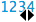I recently designed a series of data graphics for a book. When mocking up the bar charts in Photoshop, I had to calculate how tall to make the bars so they would fill the available space.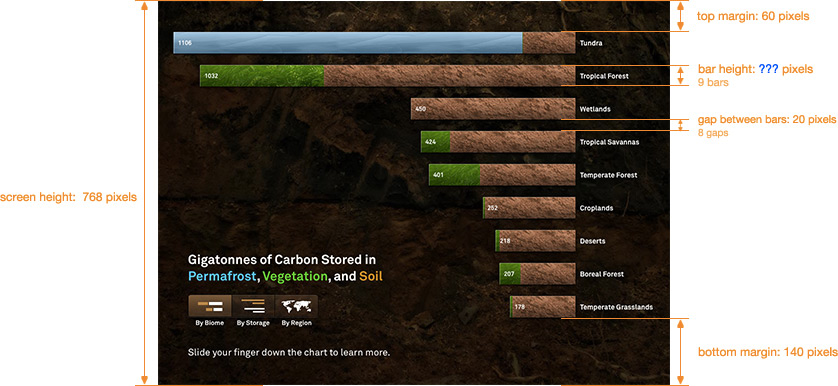I had chosen heights for the top margin, bottom margin, and gap between the bars, and I needed to know the height of the bars themselves. A conventional approach to this problem is to write out an equation: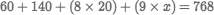and then perform algebraic manipulation to invert the equation and solve for x.

Here's a different way of finding the answer:What happened here? I typed out the expression for the total height, making a guess at the bar height. (As I typed, the tool automatically calculated the total, ignoring the words.) I saw my guess was too large (the total was more than 768), so I adjusted the bar height until the total was about right. I couldn't get it exactly right because it wouldn't divide evenly, so I added a little extra to the top margin to make everything work out.

I performed the adjustment simply by dragging horizontally on the value ("scrubbing" the number).

Notice that no algebra-style variables were used. Nor were any algebraic manipulation techniques — in a problem that is entirely about addition and multiplication, I didn't need to introduce any artificial subtraction or division.

This technique of guessing the answer and adjusting until close enough mirrors how I tend to solve problems like this in my head. To me, it feels more natural than inverting the equation.

## Double ScrubbleA friend and I recently embarked on a multi-week cross-continental adventure. We assumed we would split the expenses after the trip, so sometimes he paid for things, and sometimes I did. When we tallied it up, he had spent \$2910 and I had spent \$426. I had to figure out how much to reimburse him.

This is a simple problem, but it's not obvious. It typically would require either writing out and solving an equation: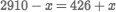or recognizing the "trick" that we have to split the difference.

Here's another way to find the answer:As before, I made a guess at how much I owed him (about \$1000). This number appeared in two places, so I selected both of them (using the standard convention for multiple selection, command-click in my case) and then scrubbed until the totals evened out. No symbolic variables were needed, nor any tricksy insights into the problem structure.

Notice that the solution reads like a pair of sentences, and you can clearly see all relevant values — how much each us spent, how much I owe him, and what the trip ended up costing each of us.

## ConnectingIn the example above, I selected two numbers so I could scrub them simultaneously. This is great for making quick calculations. But if the problem was a little more complex, and I wanted to continue to explore it later, it would be annoying to always have to remember to select both numbers.

We would like a way of indicating that the two numbers should always be the same — that they are, in fact, a single value that appears in two different places.

We can do this by connecting them:When the mouse rolls over a number, a little triangle appears in the corner. Clicking on the triangle drops down a couple of tools. Using the connection spigot, I dragged a connection from one number to the other. Notice now that when I roll over either of the numbers, they both highlight, and when I scrub either one, they both stay in sync. It is now, essentially, a single number that appears in two places.

## UnlockingBack to the problem with the bar graph: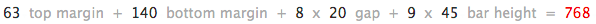As I continued to work on the design of the bar graph, I ended up reducing the margins quite a bit. I needed to find the new height for the bars.

I could simply scrub the margins to their new values, and then scrub the bar height again to bring the total back up to 768. But if I'm going to be changing the margins a lot, that could get annoying. I would prefer to indicate that the 768 should remain constant, and that the bar height should be the number that is automatically calculated.

The 768 is in red; this indicates that it is unlocked. A locked number (in black) remains fixed to whatever we set it to — it can't change on its own. An unlocked number is free to adjust itself to whatever makes the equation hold.

Here, I unlock the bar height. Now, when I adjust any of the locked numbers (even the number of bars), I can immediately see the correct bar height.## Kill x

This work is about allowing people to solve algebraic problems while working entirely with concrete numbers, instead of abstract symbols.

We are accustomed to assuming that variables must be symbols. But this isn't true — a variable is simply a number that varies. If we are able to vary numbers interactively, then we don't necessarily need to name them.

Unlocking and connecting each address a specific and common use of symbolic variables. In this case:we use x to mean, "I want to know the number that fits here, but I don't have an explicit formula for it." We conventionally use rote algebraic mechanisms to convert the equation into an explicit expression. But this is not paper, and a computer has no trouble calculating an implicitly-defined number. With a calculator like the one presented here, we can unlock any number in order to solve for it, regardless of where it appears in the equation.

In this case:we use x to mean, "There is a single number that should appear in two different places." (Note that such a number isn't necessarily a variable — when we want a constant to appear in multiple places, we typically use a symbolic name as well.) But this is not paper, and a computer has no trouble keeping track of multiple instances of an object. With a calculator like this one, we can connect numbers to make them the same, instead of having to name them.

This work is part of the Kill Math project, which is about finding concrete, tangible ways of working with quantities. Another example of such a tool is Interactive Exploration of a Dynamical System.

Scrubbable numbers also feature in Explorable Explanations, and originated (at least for me) with Ten Brighter Ideas.

Numbers that can be changed in context are also discussed in the train scheduler case study in Magic Ink.

## Exercises

I have my own ideas about the following, but I'd like to give you a chance to think about them. If you come up with ideas, write about it on your blog, or post your design on Twitter.

1. Graphs. When interactively adjusting quantities, visual representations can help enormously. The tool shown here automatically evalutes the expression you type. Could it automatically draw a graph as well? What would be graphed, and what would it look like? Try drawing a mockup, and thinking about how the interaction would work.
2. Granularity. Some numbers need to be adjusted over large ranges — you may want to go thousands in one stroke. Other numbers might need multiple decimal-point precision. The tool can't necessarily infer which is which. Can you think of a UI that could accomodate adjustments at different levels of granularity?
3. Non-unique solutions. From the tool's perspective, an unlocked number is a variable or unknown to be solved for, and the number of unlocked numbers is the dimension of the domain, or the degrees of freedom. Connecting a unlocked number introduces a constraint that might reduce the degrees of freedom. If you have too few unlocked numbers, the system is said to be over-constrained, and might not have a solution. Too many unlocked numbers means it is under-constrained, and there might be multiple solutions that fit. What would be a good UI for these situations? (Hint: if an over-constrained system has no solution, the tool must clearly indicate why, and provide an easy means to fix the problem. An under-constrained system typically has a space of solutions, often representing trade-offs between the variables, which the user might like to explore. Also think about transition states — ill-formed situations that might result while the user is entering or changing the problem.)